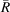International Tables for Crystallography (2006). Vol. C. ch. 9.3, pp. 774-777https://doi.org/10.1107/97809553602060000619

## Contents

• 9.3. Typical interatomic distances: metals and alloys  (pp. 774-777)
• 9.3.1. Glossary  (p. 777) | html | pdf |
• References | html | pdf |
• Figures
• Fig. 9.3.1. ( a ) The radii for CN = 12  (p. 774) | html | pdf |
• Fig. 9.3.2. ( a ) Plot of d AB versusfor the binary compounds crystallizing in hP 3 AlB 2   (p. 775) | html | pdf |
• Fig. 9.3.3. ( a ) A typical example of a next-neighbour histogram (NNH) and ( b ) the atomic environment (AE) coordination polyhedron belonging to this NNH  (p. 775) | html | pdf |
• Fig. 9.3.4. ( a ) The 23 most frequently occurring atomic environment types (AET) with their polyhedron code  (pp. 776-777) | html | pdf |